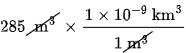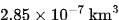×
Get Full Access to Introductory Chemistry - 5 Edition - Chapter 2 - Problem 92p
Get Full Access to Introductory Chemistry - 5 Edition - Chapter 2 - Problem 92p

×

A classroom has a volume of 285 m3. What is its volume in each unit?(a) km3(b) dm3(c)ISBN: 9780321910295 34

Solution for problem 92P Chapter 2

Introductory Chemistry | 5th Edition

• Textbook Solutions
• 2901 Step-by-step solutions solved by professors and subject experts
• Get 24/7 help from StudySoup virtual teaching assistantsIntroductory Chemistry | 5th Edition

4 5 1 241 Reviews
21
3
Problem 92P

A classroom has a volume of $$285\ \text{m}^3$$. What is its volume in each unit?

(a) $$\text{km}^3$$

(b) $$\text{dm}^3$$

(c) $$\text{cm}^3$$

Step-by-Step Solution:

Step 1 of 3

Given,  volume=.

volume in each unit = ?

(a):Thereforeinis :

==_____________________________________

Step 2 of 3

Step 3 of 3

ISBN: 9780321910295

This full solution covers the following key subjects: Volume, unit, its, classroom. This expansive textbook survival guide covers 19 chapters, and 2046 solutions. This textbook survival guide was created for the textbook: Introductory Chemistry, edition: 5. Since the solution to 92P from 2 chapter was answered, more than 5423 students have viewed the full step-by-step answer. The full step-by-step solution to problem: 92P from chapter: 2 was answered by , our top Chemistry solution expert on 05/06/17, 06:45PM. Introductory Chemistry was written by and is associated to the ISBN: 9780321910295. The answer to “?A classroom has a volume of $$285\ \text{m}^3$$. What is its volume in each unit? (a) $$\text{km}^3$$(b) $$\text{dm}^3$$ (c) $$\text{cm}^3$$” is broken down into a number of easy to follow steps, and 23 words.

Unlock Textbook Solution